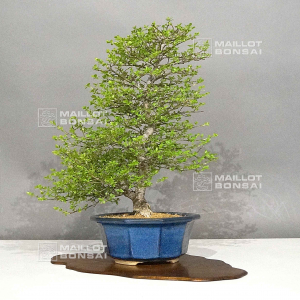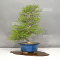##### The Japanese Bonsai specialist
Direct order Contact Help / Services Newsletter# Euvonymus sieboldianus 5050235

Euvonymus sieboldianusref. : 11928Product unavailable

###### Description

Height of the only tree: 400 mm.
Width of the branches: 370 mm.

Japanese blue sea enameled pot: 220*90 mm.

Diameter of the trunk: 30-40 mm.

Nebari: 100-110 mm. Moyogi style.

Require generous watering in summer and regular fertilization all along the dormancy period to guarantee superb autumn coloration and good fructification.

Ramified bonsai with trays, without any strong cutting wound or wire mark.

This variety has white flowers at the beginning of summer and little green canopy leaves. Particularly vigorous and perfectly adapted to bonsai cultivation.

The fruits are like hanging pink pods in autumn and let appear two red arils. The shelf is not included. Photographed in April 2023.

#bonsai 4.6 #sieboldianus 3.2 #flowering 2.9 #euvonymus 2.9 #fruiting 2.8 #summer 2.6 #autumn 2.6 #fructification 2.4 #fertilization 2.3 #photographed 2.2

Formule
(( ROUND((CHAR_LENGTH(b.article_nom)-CHAR_LENGTH(REPLACE(b.article_nom, 'sieboldianus', '')))/LENGTH('sieboldianus')) + ROUND((CHAR_LENGTH(b.article_description)-CHAR_LENGTH(REPLACE(b.article_description, 'sieboldianus', '')))/LENGTH('sieboldianus')) ) * 3.2) + (( ROUND((CHAR_LENGTH(b.article_nom)-CHAR_LENGTH(REPLACE(b.article_nom, 'euvonymus', '')))/LENGTH('euvonymus')) + ROUND((CHAR_LENGTH(b.article_description)-CHAR_LENGTH(REPLACE(b.article_description, 'euvonymus', '')))/LENGTH('euvonymus')) ) * 2.9) + (( ROUND((CHAR_LENGTH(b.article_nom)-CHAR_LENGTH(REPLACE(b.article_nom, 'autumn', '')))/LENGTH('autumn')) + ROUND((CHAR_LENGTH(b.article_description)-CHAR_LENGTH(REPLACE(b.article_description, 'autumn', '')))/LENGTH('autumn')) ) * 2.6) + (( ROUND((CHAR_LENGTH(b.article_nom)-CHAR_LENGTH(REPLACE(b.article_nom, 'summer', '')))/LENGTH('summer')) + ROUND((CHAR_LENGTH(b.article_description)-CHAR_LENGTH(REPLACE(b.article_description, 'summer', '')))/LENGTH('summer')) ) * 2.6) + (( ROUND((CHAR_LENGTH(b.article_nom)-CHAR_LENGTH(REPLACE(b.article_nom, 'bonsai', '')))/LENGTH('bonsai')) + ROUND((CHAR_LENGTH(b.article_description)-CHAR_LENGTH(REPLACE(b.article_description, 'bonsai', '')))/LENGTH('bonsai')) ) * 2.6) + (( ROUND((CHAR_LENGTH(b.article_nom)-CHAR_LENGTH(REPLACE(b.article_nom, 'fructification', '')))/LENGTH('fructification')) + ROUND((CHAR_LENGTH(b.article_description)-CHAR_LENGTH(REPLACE(b.article_description, 'fructification', '')))/LENGTH('fructification')) ) * 2.4) + (( ROUND((CHAR_LENGTH(b.article_nom)-CHAR_LENGTH(REPLACE(b.article_nom, 'fertilization', '')))/LENGTH('fertilization')) + ROUND((CHAR_LENGTH(b.article_description)-CHAR_LENGTH(REPLACE(b.article_description, 'fertilization', '')))/LENGTH('fertilization')) ) * 2.3) + (( ROUND((CHAR_LENGTH(b.article_nom)-CHAR_LENGTH(REPLACE(b.article_nom, 'photographed', '')))/LENGTH('photographed')) + ROUND((CHAR_LENGTH(b.article_description)-CHAR_LENGTH(REPLACE(b.article_description, 'photographed', '')))/LENGTH('photographed')) ) * 2.2) + (( ROUND((CHAR_LENGTH(b.article_nom)-CHAR_LENGTH(REPLACE(b.article_nom, 'particularly', '')))/LENGTH('particularly')) + ROUND((CHAR_LENGTH(b.article_description)-CHAR_LENGTH(REPLACE(b.article_description, 'particularly', '')))/LENGTH('particularly')) ) * 2.2) + (( ROUND((CHAR_LENGTH(b.article_nom)-CHAR_LENGTH(REPLACE(b.article_nom, 'cultivation', '')))/LENGTH('cultivation')) + ROUND((CHAR_LENGTH(b.article_description)-CHAR_LENGTH(REPLACE(b.article_description, 'cultivation', '')))/LENGTH('cultivation')) ) * 2.1)

## Secure payment## Delivery

Our logistic partners :04 74 55 23 48
Pépinière MAILLOT-BONSAÏ
Le Bois Frazy
01990 RELEVANT - FRANCE
on appointment